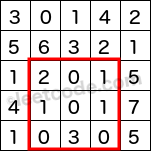# 308 Range Sum Query 2D - Mutable

### Problem:

Given a 2D matrix matrix, find the sum of the elements inside the rectangle defined by its upper left corner (row1, col1) and lower right corner (row2, col2).The above rectangle (with the red border) is defined by (row1, col1) = (2, 1) and (row2, col2) = (4, 3), which contains sum = 8.

Example:

``````Given matrix = [
[3, 0, 1, 4, 2],
[5, 6, 3, 2, 1],
[1, 2, 0, 1, 5],
[4, 1, 0, 1, 7],
[1, 0, 3, 0, 5]
]

sumRegion(2, 1, 4, 3) -> 8
update(3, 2, 2)
sumRegion(2, 1, 4, 3) -> 10
``````

Note: The matrix is only modifiable by the update function. You may assume the number of calls to update and sumRegion function is distributed evenly. You may assume that row1 ≤ row2 and col1 ≤ col2.

### Solutions:

``````public class NumMatrix {
int[][] sums;
int[][] matrix;
int m;
int n;
public NumMatrix(int[][] matrix) {
if (matrix == null || matrix.length == 0 || matrix.length == 0) {
return;
}
m = matrix.length;
n = matrix.length;
sums = new int[m + 1][n + 1];
this.matrix = new int[m][n];
for (int i = 0; i < m; i ++) {
for (int j = 0; j < n; j ++) {
update(i, j, matrix[i][j]);
}
}
}

public void update(int row, int col, int val) {
int diff = val - matrix[row][col];
for (int i = row + 1; i < m + 1; i = i + (i&(-i))) {
for (int j = col + 1; j < n + 1; j = j + (j&(-j))) {
sums[i][j] += diff;
}
}
matrix[row][col] = val;
}
private int getSum(int x, int y) {
int sum = 0;
for (int i = x; i > 0; i = i - (i&(-i))) {
for (int j = y; j > 0; j = j - (j&(-j))) {
sum += sums[i][j];
}
}
return sum;
}
public int sumRegion(int row1, int col1, int row2, int col2) {
return getSum(row2 + 1, col2 + 1) - getSum(row1, col2 + 1) - getSum(row2 + 1, col1) + getSum(row1, col1);
}
}

/**
* Your NumMatrix object will be instantiated and called as such:
* NumMatrix obj = new NumMatrix(matrix);
* obj.update(row,col,val);
* int param_2 = obj.sumRegion(row1,col1,row2,col2);
*/
``````
``````

``````